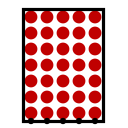#LED-Matrix

 Bibliothek: Input/Output Eingeführt: 2.2.0 Aussehen:## Verhalten

Displays a small grid of pixels, whose values are determined by the current inputs. The grid can have up to 32 rows and 32 columns.

## Pins

The interface to the component varies depending on the value of the Input Format attribute. It has three possible values.

Columns
The inputs are lined along the component's south edge, with one multibit input for each column of the matrix. Each input has as many bits as there are rows in the matrix, with the low-order bit corresponding to the southmost pixel in the column. A 1 indicates to light the corresponding pixel, while a 0 indicates to keep the pixel dim. If any of the bits for a column are either floating or error values, then all pixels in the column are lit.
Rows
The inputs are lined along the component's west edge, with one multibit input for each row of the matrix. Each input has as many bits as there are columns in the matrix, with the low-order bit corresponding to the rightmost pixel in the row. As with the Columns format, a 1 indicates to light the corresponding pixel, and a 0 indicates to keep the pixel dim. If any bits for a row are floating or error values, then all pixels in the row are lit.
Select Rows/Columns
There are two inputs on the component's west edge. The upper multibit input has as many bits as there are columns in the matrix, with the low-order bit corresponding to the rightmost column. The lower multibit input has as many bits as there are rows in the matrix, with the low-order bit corresponding to the bottom row. If any bits in either input are floating or error values, all pixels in the matrix are lit. Normally, though, a pixel at a particular row-column location is lit if the corresponding column bit in the upper input is 1 and the corresponding row bit in the lower input is 1. For example, for a 5x7 matrix, if the first input is 01010 and the second is 0111010, then the second and fourth columns are lit for the second, third, fourth, and sixth rows; the result appears to be a pair of exclamation points. (This input format may seem unintuitive, but LED matrixes are sold commercially with exactly this interface. Lite-On sells such components, for example.)

## Attribute

Input Format (read-only after component is created)
Selects how the pins correspond to pixels, as outlined above.
Matrix Columns
Selects how many columns are in the matrix, which may range from 1 up to 32.
Matrix Rows
Selects how many rows are in the matrix, which may range from 1 up to 32.
On Color
Selects the color of a pixel when it is lit.
Off Color
Selects the color of a pixel when it is dim.
Light Persistence
When this is other than 0, a pixel that is lit remains lit for the given number of clock ticks after the component's inputs indicate that the pixel should become dim.
Dot Shape
The square option means that each pixel is drawn as a 10x10 square, filling the component with no gaps between pixels. The circle option means that each pixel is drawn as a diameter-8 circle, with gaps between each circle. The circle option is more difficult to interpret, but it more closely approximates the off-the-shelf LED matrix components.

Keines.

## Verhalten des Textwerkzeugs

Keines.

Zurück zur Bibliotheksreferenz